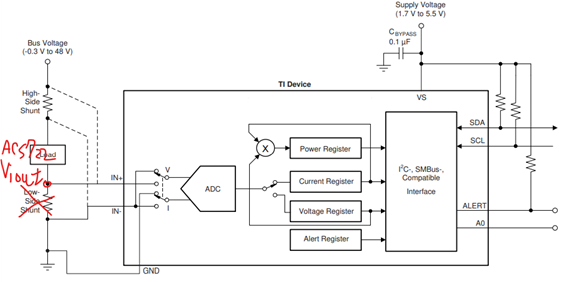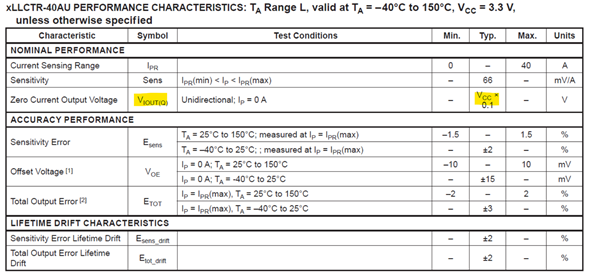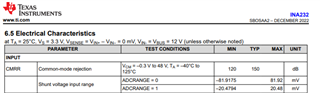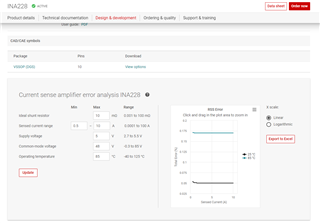If you have a related question, please click the "Ask a related question" button in the top right corner. The newly created question will be automatically linked to this question.

# INA232: Rsense resistor change to CT

Part Number: INA232

Hi Team,

The customer of INA232 questioned that the resolution of the 30A range may be poor at light load, and the power consumption will be too much at full load.

Can the Rsense part be changed to a CT IC? thanks.

• Hi Rock,

Do you have a part number for the CT IC? Also, what is the low current range needing to be measured for this system?

It sounds like you will still need an external shunt so that the INA232 can measure a voltage drop, but additional info about the CT and how the customer plans to implement it such as a schematic would be helpful.

Thanks,

Cierra

• Hi Cierra,

The customer would like to consider that OCP uses a one-way 40A CT IC, and CT Viout is connected to INA232 to replace Rsense.• Hi Rock,

This ACS722 is a Hall-effect current sensor, not a current transformer (CT has a current output). Hall-effect current sensors and shunt-based current sensors (ex: INA232) operate similarly, they measure a current and provide a proportional voltage output.

I would not recommend feeding the output of the ACS722 to the input of INA232, there is no benefit to feed one current sensor to another current sensor in this way.

It also will not work because INA232 has a max Vsense of 81.92mV. Assuming VCC = 3V for the ACS722, the zero current output voltage will be 0.3V or 300mV, based on the spec you have highlighted above.You should determine whether you need either a Hall-effect or shunt-based current sensor. Please see this TIPL training to determine which option is best for you: 5.1 Introduction to magnetic current sensing

If the customer does not require isolation, I recommend you use a shunt-based current sensor.

1. What is the minimum current you want to measure? What is considered 'light load'? 1mA, 10mA, 100mA?

2. What is the accuracy % desired at minimum current?

3. How much power can customer allow to dissipate through the Rshunt resistor?

For example,

if Imax = 30A, and INA232 Vsense max ~= 81mV, we can choose Rshunt = 2.7mOhm

Power dissipated = I^2 * R = (30A)^2 * 2.7mOhm = 2.46W

Assume minimum current to be measured Isense = 200mA

When Isense = 200mA, Rsense = 2.7mOhm, then Vsense will be 0.54mV or 540uV

Voffset max = 50uV (from INA232 datasheet)

So there will be 50uV/540uV * 100% = 9.23% error due to offset for this case (There are additional sources of error as well, at lower currents offset error tends to dominate. See TIPL training: 3.1 Introduction to different error sources

You can decrease Rshunt to lower the power dissipated, but the error due to offset will increase. Additionally, you can consider some digital power monitors with higher resolution and accuracy such as the INA228. There is a total error calculator provided on this product page that will allow you to modify the shunt resistance and quickly calculate the total error.However, if you decide to use a Hall-effect current sensor, I would recommend TI's TMCS1107 which is the same pinout as the ACS722 SOIC-8 package. TMCS1101 is another option with the same SOIC-8 pin-out, and higher accuracy. Please note the ACS has a ratiometric output, TMCS devices have a non-ratiometric output.

Thanks,

Cierra